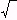edHelper subscribers - Create a new printable

Sample edHelper.com - Functions Worksheet

 Name _____________________________Date ___________________
Relations and Functions

1.   write the graph of
f(x)  =
|
 x
|
with a horizontal compression by a factor of 1/4
2.   write the graph of
f(x)  =
 x 2
with a reflection across the y-axis
3.   write the graph of
f(x)  =( x )
with a vertical translation 9 units to the left
4.   write the graph of
f(x)  =
 x 2
with a vertical translation 7 units up
5.   write the graph of
f(x)  =
 x 2 + 3
with a reflection across the x-axis
6.   write the graph of
f(x)  =
 3 ( x - 4 ) 2
with a vertical translation 9 units to the right and a vertical compression by a factor of 9/11
7.   write the graph of
f(x)  =
4 |
 x
|
with a horizontal compression by a factor of 1/4, a vertical translation 4 units down, and a reflection across the x-axis
8.   write the graph of
f(x)  =
|
 - x
|
with a horizontal stretch by a factor of 6 and a vertical stretch by a factor of 3
9.   write the graph of
f(x)  =
 x 2
with a horizontal stretch by a factor of 9
10.   write the graph of
f(x)  =
3( x )
-  2
with a vertical compression by a factor of 5/8 and a reflection across the x-axis
11.   write the graph of
f(x)  =
 x 2
with a reflection across the y-axis, a vertical stretch by a factor of 6, and a vertical translation 2 units up
12.   write the graph of
f(x)  =
|
 x
|
with a reflection across the x-axis, a horizontal stretch by a factor of 4, and a reflection across the y-axis
13.   write the graph of
f(x)  =( x + 3 )
with a vertical compression by a factor of 2/5 and a vertical translation 5 units down
14.   write the graph of
f(x)  =
 2 x 2
with a horizontal compression by a factor of 1/3

Sample
This is only a sample worksheet.

edHelper subscribers - Create a new printable